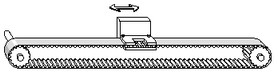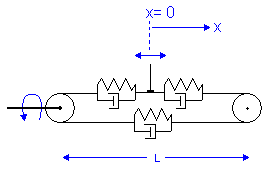﻿ 20-sim webhelp > Library > Iconic Diagrams > Mechanical > Rotation > Gears > timingbelt

# TimingBelt.emx

## Library

Iconic Diagrams\Mechanical\Rotation\Gears

Iconic Diagrams\Mechanical\Translation\Transmission

## Use

Domains: Continuous. Size: 1-D. Kind: Iconic Diagrams (Rotation/Translation).

## Introduction

This models represents a timing belt, used for linear positioning.It has a rotating pulley which drives the the belt and clamp. The timing belt is modeled by a series of spring damper elements that convey the rotation of the pulley to a clamp translation. Because the output position is moving, stiffness and damping values are not constant.The stiffness for a piece of belt can be expressed as:

k = E*A / l

with

E  = Modulus of elasticity {N/m2}

A = Belt area {m2}

l = belt length {m}

If the belt is sufficiently pre-tensioned, the stiffness experienced at the clamp can be expressed as the combination of three individual belt parts:

k = E*A/(0.5*l+x) + 1/(  1 / E*A/(0.5*l - x)  + 1 / E*A / l  )

which can be rewritten to:

k = E*A*( 1/(0.5*l+x) + 1/(1.5*l - x) )

The stiffness approaches infinity as the clamp moves to the driven pulley (x = -l/2) and has a minimum value when the clamp moves to the other pulley (x = 0.5*l). The minimum stiffness is equal to:

k = 2*E*A / l

The belt position is determined by the internal variable x. For x = 0 the clamp is in the middle. When the position crosses the driven pulley, i.e.

x < - belt_length/2

the simulation is stopped: "Error: clamp position larger than belt end!". When the position crosses the other pulley, i.e.

x > belt_length/2

the simulation is also stopped, "Error: clamp position smaller than belt start!".

## Description - Default

In this model the minimum stiffness is used, based on an output position at a length L of the driven pulley.

k = 2*E*A / l

## Description - VariableStiffness

In this model a variable stiffness is used equal to:

k = E*A*( 1/(0.5*l+x) + 1/(1.5*l - x) )

Take care not to let the clamp get too close to the driven pulley, because the stiffness will then grow to infinity!

## Interface

 Ports Description p_rot p_trans Rotation port. Translation port. Causality p_rot notequal p_trans Parameters radius d E A l pinion gear pitch radius [m] damping N.s/m] Modulus of elasticity [N/m2] A = Belt area [m2] belt length [m] Variables x clamp position, abs(x) <  belt length/2 Initial values x_initial Initial clamp position, abs(x_initial) < belt length/2